# A Modified Jellyfish Search Optimizer with Opposition Based Learning and Biased Passive Swarm Motion

A Modified Jellyfish Search Optimizer with Opposition Based Learning and Biased Passive Swarm Motion
School of Computer Science, University of Petroleum and Energy Studies, Dehradun 248007, India

Corresponding Author Email:
jiten_rajpurohit@yahoo.com
Page:
577-584
|
DOI:
https://doi.org/10.18280/isi.260608
12 October 2021
|
Accepted:
3 December 2021
|
Published:
27 December 2021
| Citation

OPEN ACCESS

Abstract:

Jellyfish Search Optimizer (JSO) is one of the latest nature inspired optimization algorithms. This paper aims to improve the convergence speed of the algorithm. For the purpose, it identifies two modifications to form a proposed variant. First, it proposes improvement of initial population using Opposition based Learning (OBL). Then it introduces a probability-based replacement of passive swarm motion into moves biased towards the global best. OBL enables the algorithm to start with an improved set of population. Biased moves towards global best improve the exploitation capability of the algorithm. The proposed variant has been tested over 30 benchmark functions and the real world problem of 10-bar truss structure design optimization. The proposed variant has also been compared with other algorithms from the literature for the 10-bar truss structure design. The results show that the proposed variant provides fast convergence for benchmark functions and accuracy better than many algorithms for truss structure design.

Keywords:

swarm intelligence, nature inspired optimization, metaheuristic algorithms

1. Introduction

Optimization algorithms can be classified in several ways. On the basis of use of random numbers, there are Deterministic and Stochastic Algorithms. Deterministic algorithms provide exact optimum solution every time they run but they are not applicable to complex non-linear problems. Stochastic algorithms are solutions to such problems. Stochastic optimization algorithms deploy heuristic search methods. Based on the number of search particles deployed, they can be Single Solution based or Population based. They are also referred as Metaheuristic Algorithms as these algorithms do not require any information about the nature of the search space and can be implemented on any type of optimization problem. Search methods deployed in these algorithms are often inspired by one or more natural phenomenon or real life process giving them the popular identity as Nature Inspired Algorithms. Some salient features of nature inspired algorithms are listed below:

(1) They use random numbers and thus may provide a different solution every time they execute.

(2) The solution may not be the exact optimum but in most cases, it is an acceptable solution.

(3) Information of the search space is not required. Only the objective function and feasible ranges of the variables are needed.

(4) Search agents deployed in the feasible space move to improve their location.

(5) Location of the global best agent is the output of the algorithm.

There is no fixed deterministic rule to move the agents in the space, so each algorithm uses algorithm specific methods to search better values. These search methods involve very high number of evaluations of the objective function and thus require computer based calculations. With the advance of computing capabilities, these algorithms have been able to adopt new sophisticated methods and the number of new nature inspired algorithms has increased many folds in the last few decades. Few popular latest algorithms are Grey Wolf Optimizer , Whale Optimization Algorithm , Water Strider Algorithm  and Black Widow Optimization Algorithm .

This paper proposes a modified variant of a recently introduced nature inspired algorithm i.e. Jellyfish Search Optimizer (JSO) . Two modifications are proposed in the basic JSO to form the modified variant.

One of the modifications is Opposition based Learning (OBL). OBL is a learning operator widely used in nature inspired optimization algorithms and other computational intelligence methods. OBL generates a point that is opposite of the given point. The generated point is opposite with respect to the upper and lower limits of the variable. An opposite member O’ for a point O with upper and lower limits UL and LL respectively is denoted in Eq. (1).

$O^{\prime}=L L+U L-O$                     (1)

OBL based learning methods vary on further use of the opposite members, but generally a selection is performed between O and O’ to select the better one and drop the other one.

The proposed variant is then validated by solving 30 benchmark functions and the 10-bar truss structure design optimization problem. Rest of the article is structured as follows: Section 2 explains the basic steps of the working of JSO. Section 3 investigates the literature for similar modifications done on other algorithms. Section 4 explains the modifications and the proposed variant. Section 5 presents the experimental results over benchmark functions and discussion. Section 6 shows the proposed variant’s implementation to solve the 10-bar truss design problem. Finally, Section 7 concludes the paper while providing future direction of the work.

2. Jellyfish Search Optimizer

JSO is inspired by the survival behavior exhibited by Jellyfish in the waters.

Like any other population based nature inspired optimization algorithm, JSO also has three basic steps of Initialization, Generations and Termination. All these steps are explained further.

Step 1: Initialization

JSO uses logistic map to start with an improved set of population. A logistic sequence is generated using Eq. (2).

$C_{j+1}=4 \times C_{j}\left(1-C_{j}\right)$                   (2)

where, j=1 indicates dimension of the problem and initial value for the sequence $C_{0}=\operatorname{rand}(0,1)$ but $C_{0} \neq\{1|0.75| 0.5 \mid 0.25\}$. Eq. (2) will create a sequence of ND numbers in the range (0,1).

Then the members of the population are initialized using Eq. (3).

$X_{i, j}=L L_{j}+\left(U L_{j}-L L_{j}\right) \times C_{j}$                  (3)

where, i=0 the population size.

Step 2: Generation Loops

Each generation updates the location of each member by moving it in the space. A control function c(g) controls the type of movements. For each member, the value of c(g) is calculated by Eq. (4).

$c(g)=\mid\left(1-\frac{g}{G_{\max }}\right) \times(2 \times$ rand $-1) \mid$                   (4)

where, g is the generation count, Gmax is the maximum allowed generations and rand is a random number from (0,1).

Based upon the value of c(g), each jellyfish can have two types of movements i.e. ocean current movement and swarm motion.

A jellyfish observes ocean current movement if c(g)≥Cin. Ocean currents contribute to exploration capabilities of the algorithm. Cin is the coefficient that controls the degree of exploration and exploitation of the algorithm. Eq. (5) expresses the ocean current movement.

$x_{i}(g+1)=x_{i}(g)+rand \times\left(x_{\text {best }}- rand \times \beta \times \frac{\sum_{k=1}^{N P} x_{k}}{N P}\right)$              (5)

where, xbest is the member of the population with the best objective value and β is called the distribution coefficient.

If a jellyfish is not observing an ocean current movement, then it undergoes a swarm motion. Swarm motion provides the exploitation power to the algorithm. Swarm motion can be of either Active or Passive type.

If rand>(1-c(g)) then it performs passive motion, otherwise active motion. Eq. (6) and Eq. (7) express these motions respectively.

$x_{i}(g+1)=x_{i}(g)+(U L-L L) \times \gamma \times$ rand                 (6)

where, $\gamma>0$ is the motion coefficient. Passive swarm motion enables a jellyfish to exploit its surroundings for searching better locations.

$x_{i}(g+1)=\begin{cases}x_{i}(g)+\left(x_{k}-x_{i}(g)\right) \times \text { rand, } &\text{if}\,\, f\left(x_{k}\right) \geq f\left(x_{i}(g)\right) \\ x_{i}(g)+\left(x_{i}(g)-x_{k}\right) \times \text { rand } & \text { Otherwise }\end{cases}$                      (7)

where, k is a randomly chosen index between 1 to NP and f(x) denotes the objective function value of x. Active swarm motion depicts the movement of a jellyfish towards a better jellyfish in the population. Figure 1 shows the logical sequence of steps for each member in a generation loop.

The updated location of a jellyfish is checked for violation of limits UL and LL. In case of violation, the location is updated to fall inside the limits again. This update is done on the opposite side of the limit. If an updated member violates the upper limit ULj by $\Delta_j$ and is located at ($UL_j+\Delta_j$) then it is updated to a new location at ($LL_j+\Delta_j$) in dimension j.

Step 3: Termination

A predefined termination condition is checked after each generation. In all the experiments of this paper, a maximum number of generations (Gmax) is set as the termination condition.

3. Literature Review

This paper includes Opposition Based Learning (OBL) to improve the convergence speed of JSO. OBL provides an improved initial population to start the generation loops . This initial advantage most of the times affects the quality of the ultimate solution. Literature is rich with the use of OBL as a tool to improve nature inspired algorithms. Most of the work in the literature suggests that OBL is implemented with one or more other tools for enhancement. A part of the literature is reviewed and the findings are presented in Table 1. Details of the additional tools with the OBL are also indicated.

## 1.pngFigure 1. Types of movement inside generation loops

Table 1. Findings of the literature review

 Year Algorithm Implementation Details Reference 2021 Elephant Herding Optimization To overcome the slow convergence of the algorithm due to lack of exploitation component, OBL and position update operator from Sine-cosine algorithm are implemented.  2021 Class Topper Optimization Along OBL, the paper uses fractional order to update positions. This combination provides a balance between exploitation and exploration.  2020 Firefly Algorithm OBL along with three methods adopted from the working of Dragonfly Algorithm are used to modify Firefly Algorithm to avoid local optimum.  2020 Salp Swarm Algorithm OBL and a new local search strategy are used to improve Salp Swarm algorithm for feature selection.  2020 Crow Search Algorithm OBL is used to enhance initial population. A cosine function is used to accelerate exploration to avoid local optima.  2019 Monarch Butterfly Optimization Algorithm OBL generates an opposite population. Better of the two opposite members is then selected to continue. A Random local Perturbation is then used to improve the migration operator.  2019 Multifactorial Evolutionary Algorithm Integration of OBL and DE is used to improve the convergence of the algorithm. Different search territories of DE and OBL along with their complementary nature and the simulated binary crossover are the motivation for the algorithm.  2019 Dragonfly Algorithm Selection between a member of the population and its opposite member is performed throughout the execution to continuously improve the solution. The variant has been implemented on multi-level thresholding image segmentation.  2019 Shuffled Complex Evolution OBL with an improved competition complex evolution strategy is used to improve accuracy, efficiency and population diversity of the algorithm.  2018 Grasshopper Optimization Algorithm First phase of the enhancement generates opposites of the initial population. Second phase improves half of the population in generation loops using OBL. 
4. Proposed Modified JSO (MJSO)

Following are two modifications proposed in the structure of basic JSO.

OBL in initialization

OBL is implemented as a tool to improve initial population. Summary of literature review in Table 1 is evident that OBL in initialization step is a proven method of improving convergence. After initialization, using Eq. (3), a set of opposite members X’ is generated for the initial set of population X, temporarily having 2NP members. Best NP members are then selected from the set ($X \cup X^{\prime}$). Figure 2 visualizes this update for 2- dimensional Rastrigin function with initial population size of 10. Figure 2(a) shows the initial population with red circled markers and the opposite member generated for each member as green circled markers. Figure 2(b) shows the best 10 members selected from the union of both the populations.

Improved Passive Swarm Motion

Ocean currents in JSO are aimed at exploration while swarm motion targets exploitation. Coefficient Cin trades off the degree of both these capabilities. The time control c(g) of Eq. (4) allows exploratory ocean currents during the initial phase of the execution. As the execution advances, ocean current movements are replaced by swarm motion to increase the degree of exploitation. Among swarm motion, active swarm motion moves keep on increasing with the generation count while passive motion keeps on suppressing. Figure 3 demonstrates the number of all the three types of moves for a typical run of 500 generations in a population of 100 for Cin=0.5. Exploitation aims to converge the algorithm.

The movement of jellyfish in passive swarm motion enables it to search its surrounding for better locations. This search is unbiased hence has a low probability of finding better values and thus contributing least to the whole purpose of exploitation. The proposed modification is to add a directional bias to passive swarm motion to enhance convergence. The direction of this bias is kept towards the global best jellyfish xbest. Passive swarm motion is not removed completely but a probability-based method has been deployed to replace some passive swarm motion into biased moves towards global best. A coefficient called Convergence Bias (CB) is introduced to control the magnitude of the bias. Mathematically, the passive swarm motion of Eq. (6) is replaced by Eq. (8). Figure 4 demonstrates both these movements.

$x_{i}(g+1)=\left\{\begin{array}{l}x_{i}(g+1)=x_{i}(g)+\left(x_{\text {best }}-x_{i}\right) \times \text { rand, } &\text { if } C_{B}>\text { rand } \\ x_{i}(g+1)=x_{i}(g)+(U L-L L) \times \gamma \times \text { rand, } &\text { Otherwise }\end{array}\right.$                   (8)

## 2a.png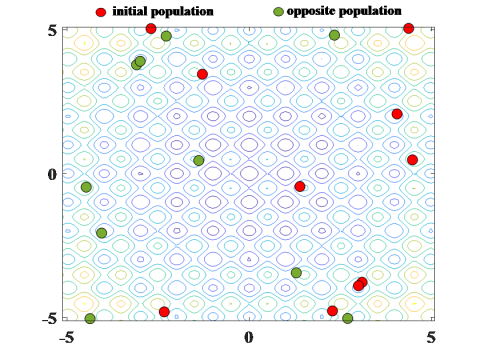(a)

## 2b.png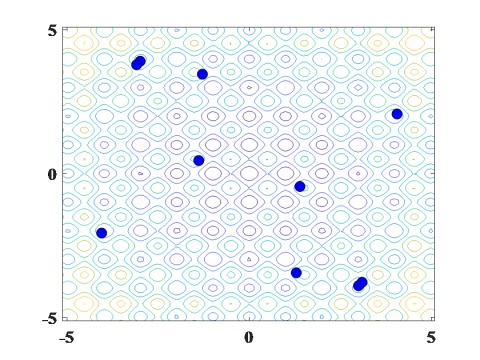(b)

Figure 2. (a) initial population and its opposite population (b) best members from the union of both the population sets

## 3a.png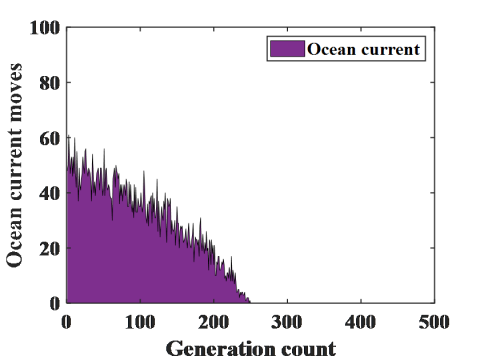(a)

## 3b.png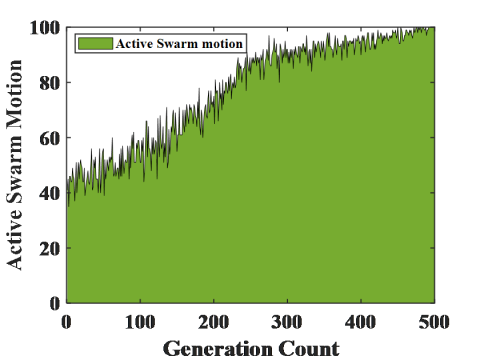(b)

## 3c.png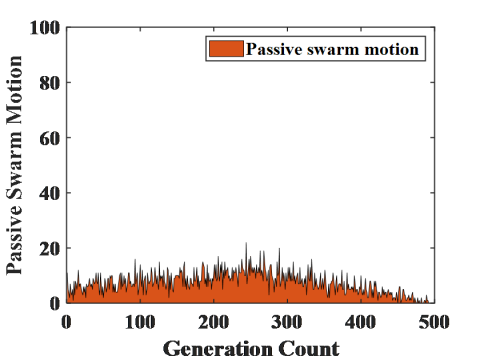(c)

Figure 3. Number of (a) ocean current (b) active swarm and (c) passive swarm movements in a typical run of 500 generations for NP=100 and Cin=0.5

## 4.png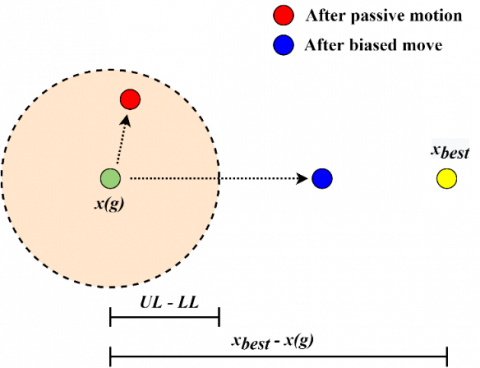Figure 4. Movement of a jellyfish x(g) in passive swarm motion and proposed biased move

5. Experimental Result and Analysis for Benchmark Functions

The proposed modified variant (MJSO) has been tested over a comprehensive set of 30 diverse benchmark minimization test functions . The set includes both unimodal (f1 to f9) and multimodal (f10 to f30) functions. Table 2 contains experimental environment details and parameter settings.

Table 2. Experimental environment and parameter settings

 Experimental Environment Tool MATLAB R2021a Processor Intel(R) Core(TM) i7-8565U CPU @ 1.80GHz RAM 16 GB Operating System Windows 10 Parameter Settings JSO coefficients Cin, γ, β 0.5, 0.1, 3 Population size (NP) 50 Maximum generations (Gmax) 500 CB for MJSO 0.25

Due to the inherent property of randomization of the algorithms, all experiments were repeated for 30 runs. Best and mean objective function values obtained for f1 to f30 along with the standard deviation are provided in Table 3. Necessary details about the functions are also included in Table 3. The source  can be referred for more details. Best values among the two algorithms are written in bold text. Mean values are used as the primary criteria for further analysis. Success Rate (SR) is defined as the percentage of functions for which the algorithm performs sole or the joint best. SR is calculated by Eq. (9).

$S R=\frac{\text { Number of functions the algorithm performs best }}{\text { Number of total functions }} \times 100$                   (9)

JSO and MJSO succeed to be the best algorithms for 18 and 27 functions respectively obtaining SRs of 60% and 90%. For unimodal functions, the algorithms succeed for 4 and 8 functions respectively while for multimodal functions they succeed for 14 and 19 functions. Table 3 provides sufficient evidence to establish that MJSO outperforms JSO for unimodal as well as multimodal functions. Although for majority of functions, both algorithms are successful to find optimum and hence their difference is not visible in Table 3. To visualize convergence speeds, convergence graphs are plotted for all such functions f1, f3, f4, f10, f11, f12, f15, f18, f19, f20, f21, f22, f23, f24 and f25 in Figure 5. The figure manifests that MJSO provides faster convergence for most of the functions that could not be differentiated in Table 3.

6. 10-Bar Truss Design Optimization for Continuous Design Variables

Truss structures  are a rich class of optimization problems to act as benchmarks for algorithms. Literature has a good number of algorithms implemented to solve problems from the set. The 10-bar truss optimization problem has been optimized by Genetic Algorithm , Particle Swarm Optimization [18, 19], Harmony Search [20, 21], Artificial Bee Colony  and Teaching Learning based Optimization . A truss is a combination of bars connected at a few joints and is designed to handle certain limits of deflection and stress. Weight of a bar in the truss is a function of its cross section area. After fixing the geometry of the truss, cross section areas of bars become the variables for the optimization problem. Objective of the optimization is to minimize total weight of all the bars put together while stress and deflection limits act as the constraints. Eq. (10) expresses the objective function.

Table 3. Best, mean and standard deviation values of benchmark test functions for JSO and MJSO

 Function symbol Function name JSO MJSO Dimension Best Mean Std Best Mean Std f1 Stepint 5 0.00E+00 0.00E+00 0.00E+00 0.00E+00 0.00E+00 0.00E+00 f2 Quartic 30 4.68E-05 3.33E-04 1.67E-04 8.99E-05 3.51E-04 1.78E-04 f3 Beale 2 0.00E+00 0.00E+00 0.00E+00 0.00E+00 0.00E+00 0.00E+00 f4 Easom 2 -1.00E+00 -1.00E+00 0.00E+00 -1.00E+00 -1.00E+00 0.00E+00 f5 Colville 4 1.88E-11 1.34E-06 2.26E-06 1.49E-15 2.19E-08 9.35E-08 f6 Trid6 6 9.54E-20 2.52E-15 9.36E-15 4.36E-25 2.14E-19 9.07E-19 f7 Powell 24 1.76E-26 7.50E-08 2.97E-07 2.67E-32 5.87E-10 1.90E-09 f8 Rosenbrock 30 3.85E-04 1.19E-01 2.55E-01 1.44E-04 6.97E-03 1.24E-02 f9 Dixon-Price 30 6.26E-03 4.51E-02 4.06E-02 1.66E-03 2.92E-02 3.72E-02 f10 Foxholes 2 9.98E-01 9.98E-01 8.25E-17 9.98E-01 9.98E-01 4.12E-17 f11 Branin 2 3.98E-01 3.98E-01 0.00E+00 3.98E-01 3.98E-01 0.00E+00 f12 Booth 2 0.00E+00 0.00E+00 0.00E+00 0.00E+00 0.00E+00 0.00E+00 f13 Rastrigin 30 1.13E-06 3.61E-02 1.90E-01 1.07E-07 1.22E-04 3.64E-04 f14 Schwefel 30 -1.16E+04 -8.98E+03 1.44E+03 -1.21E+04 -1.06E+04 1.06E+03 f15 Michalewicz2 2 -1.80E+00 -1.80E+00 9.03E-16 -1.80E+00 -1.80E+00 9.03E-16 f16 Michalewicz5 5 -4.69E+00 -4.67E+00 1.93E-02 -4.69E+00 -4.65E+00 5.84E-02 f17 Michalewicz 10 10 -9.62E+00 -9.50E+00 1.21E-01 -9.66E+00 -9.41E+00 1.83E-01 f18 Shubert 2 -1.87E+02 -1.87E+02 3.68E-11 -1.87E+02 -1.87E+02 2.11E-14 f19 GoldStein-Price 2 3.00E+00 3.00E+00 1.35E-15 3.00E+00 3.00E+00 1.49E-15 f20 Kowalik 4 3.07E-04 3.07E-04 8.52E-09 3.07E-04 3.07E-04 2.48E-17 f21 Shekel5 4 -1.02E+01 -1.02E+01 1.78E-11 -1.02E+01 -1.02E+01 5.96E-15 f22 Shekel7 4 -1.04E+01 -1.04E+01 4.66E-16 -1.04E+01 -1.04E+01 6.60E-16 f23 Shekel10 4 -1.05E+01 -1.05E+01 1.55E-15 -1.05E+01 -1.05E+01 9.90E-16 f24 Powersum 4 3.89E+00 3.89E+00 2.81E-15 3.89E+00 3.89E+00 1.91E-15 f25 Hartman6 6 -3.32E+00 -3.32E+00 1.46E-11 -3.32E+00 -3.32E+00 8.08E-16 f26 Penalized 30 1.45E-11 3.81E-10 6.44E-10 6.35E-12 2.40E-10 4.67E-10 f27 Penalized2 30 6.80E-11 8.13E-09 1.05E-08 2.74E-11 7.12E-09 2.36E-08 f28 Fletcher Powell2 2 0.00E+00 1.97E-17 7.59E-17 0.00E+00 5.38E-26 2.37E-25 f29 Fletcher Powell5 5 2.09E-13 2.39E+01 1.24E+02 1.54E-18 5.95E+00 1.82E+01 f30 FletcherPowell10 10 1.82E-10 4.04E+01 1.30E+02 5.14E-17 1.41E+01 5.09E+01 SR 60% 90%

## 5a.png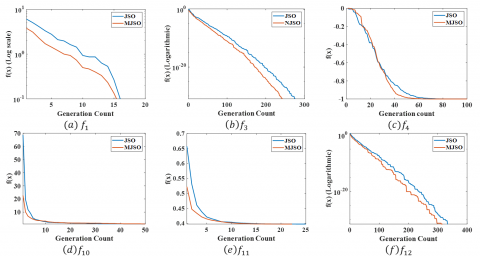## 5b.png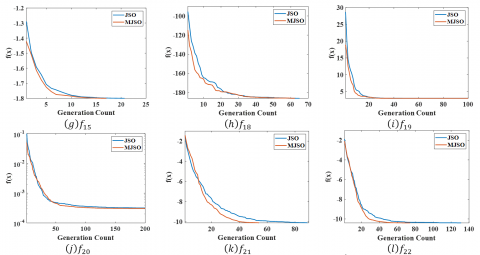## 5c.png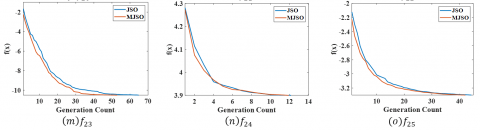Figure 5. (a)-(o) Comparison of convergence speeds of JSO and MJSO for selected functions

Minimize $W=\sum_{b=1}^{n} c_{b} l_{b} d_{b}$              (10)

Subject to:

$\sigma_{L L} \leq \sigma_{b} \leq \sigma_{H H}$                 (10.1)

$\delta_{L L} \leq \delta_{j} \leq \delta_{H H}$                     (10.2)

$c_{L L} \leq c_{b} \leq c_{H H}$                      (10.3)

where, b denotes the bar identifier and b = 1 to n. Cross section area, length and density of the material of bar b are denoted by $c_{b}, l_{b}$, and $d_{b}$ respectively. Stress of bar b and deflection at a joint j are denoted by $\sigma_{b}$ and $\delta_{j}$ while $\sigma_{L L}$ and $\sigma_{H H}$ represent lower and upper limits of the allowed ranges of all the three constraints in Eqns. (10.1)-(10.3).

Stress penalty for a bar b is computed by Eq. (11).

$\varphi_{\sigma}^{b}=\left\{\begin{array}{lr}0 & \text { if } \sigma_{L L} \leq \sigma_{b} \leq \sigma_{H H} \\ \left|\frac{\left(\sigma_{H H}-\sigma_{L L}\right)-\sigma_{b}}{\left(\sigma_{H H}-\sigma_{L L}\right)}\right| & \text { otherwise }\end{array}\right.$                         (11)

Cumulative stress penalty for a design d is calculated by Eq. (12).

$\varphi_{\sigma}^{d}=\sum_{b=1}^{n} \varphi_{\sigma}^{b}$                        (12)

Deflection penalty in each direction x, y and z at a joint j is calculated by Eq. (13).

$\varphi_{\delta(x, y, z)}^{j}=\left\{\begin{array}{lr}0 & \text { if } \delta_{L L} \leq \delta_{j(x, y, z)} \leq \delta_{H H} \\ \frac{\left(\delta_{H H}-\delta_{L L}\right)-\delta_{j(x, y, z)}}{\left(\delta_{H H}-\delta_{L L}\right)} & \text { otherwise }\end{array}\right.$                     (13)

Cumulative deflection penalty for a design d of the structure with m joints is given by Eq. (14).

$\varphi_{\delta}^{d}=\sum_{i=1}^{m}\left(\varphi_{\sigma x}^{m}+\varphi_{\sigma y}^{m}+\varphi_{\sigma z}^{m}\right)$                   (14)

Cumulative penalty for a design d is expressed as given in Eq. (15).

$P^{d}=\left(1+\varphi_{\sigma}^{d}+\varphi_{\delta}^{d}\right)^{\varepsilon}$                (15)

where, $\varepsilon$ is the penalty exponent. The penalized objective function for minimum weight for a design d now becomes as expressed in Eq. (16).

$F^{d}=W \times P^{d}$                 (16)

10-bar truss is a cantilever truss with 6 joints. Figure 6 shows geometry of a 10-bar truss structure. The figure shows 10 bars A-J each of length 360 inches. The cross section area for each bar is between 0.1 in2 and 35 in2. The material density (weight/volume) and elasticity are 0.1 lb/in3 and 107 psi respectively. Maximum allowed stress violation for a bar is ±25 ksi and maximum allowed deflection at a joint is ±2 in.

## 6.pngFigure 6. Geometry of 10-bar truss structure

The experiments for 10-bar truss design optimization were conducted for JSO and MJSO for 1000 generations and with all other parameter settings of Table 2. Table 4 contains the results. The table displays the best weight Wbest obtained and the cross section areas of all the 10 bars for Wbest for JSO and MJSO. It also contains average weight Wavg, standard deviation of weights Wstd and the number of objective function evaluation NFE. The table also inherits the results for the same problem from the literature as reported in the reference . It can be observed that on the criterion of Wbest MJSO outperforms GA, HPSO, SAHS and TLBO. Average weight is given for only three algorithms in the literature and MJSO outperforms PSO.

A comparative convergence graph containing average values of penalized weights for JSO and MJSO is shown in Figure 7. It is evident from the figure that MJSO continuously provides better values than JSO making it a variant that is capable of providing better results at every stage of execution.

## 7.png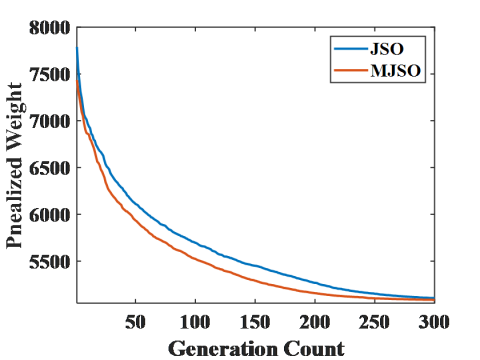Figure 7. Convergence graph for penalized weight of 10-bar truss design for JSO and MJSO

Table 4. Comparative results for 10-bar truss design optimization for JSO, MJSO and literature

 Variable A B C D E F G Cross section area JSO 30.5030 0.1005 23.1995 15.1241 0.1000 0.5468 21.2243 MJSO 30.5821 0.1000 23.0505 15.1876 0.1000 0.5528 21.0851 GA  28.920 0.100 24.070 13.960 0.100 0.560 21.950 PSO  29.999 0.100 23.268 15.129 0.100 0.554 21.232 HS  30.150 0.102 22.710 15.270 0.102 0.544 21.560 HPSO  30.704 0.100 23.167 15.183 0.100 0.551 20.978 ABC  30.548 0.100 23.180 15.218 0.100 0.551 21.058 SAHS  30.394 0.100 23.098 15.491 0.100 0.529 21.189 TLBO  30.6684 0.1000 23.1584 15.2226 0.1000 0.5421 21.0255 Variable H I J Wbest Wavg Wstd NFE(×103) Cross section area JSO 7.4820 0.1000 21.4063 5061.0814 5065.1659 7.0011 50 MJSO 7.4775 0.1000 21.5475 5060.9112 5064.9284 6.5316 50 GA  7.690 0.100 22.090 5,076.31 - - 15 PSO  7.454 0.100 21.670 5,059.85 5067.51 17.509 10.19 HS  7.541 0.100 21.450 5,057.88 - - 20 HPSO  7.460 0.100 21.508 5,060.92 - - 12.5 ABC  7.463 0.100 21.501 5,060.88 - - 500 SAHS  7.488 0.100 21.342 5,061.42 5061.95 0.71 7.081 TLBO  7.4654 0.1000 21.4660 5,060.973 5064.808 6.3707 13.767
7. Conclusion and Future Work

The experiments conducted for JSO and the proposed MJSO are sufficient to establish that MJSO outperforms JSO for unimodal, multimodal and truss design optimization. Opposition based learning is a proven tool to improve the efficiency of population based algorithms. Any bias towards global best is expected to improve convergence for unimodal functions but the proposed variant identifies passive swarm motion that can be replaced for biased movements without affecting the convergence for multimodal functions as well. Furthermore, few directions for future work are suggested below:

(1) To check and possibly improve the performance of JSO on multi-objective problems.

(2) The proposed work tests biased moves in the passive swarm motion step of JSO. Similar bias can be tested in active swarm motion and the ocean current.

(3) JSO can be hybridized with other nature-inspired algorithms to develop a hybrid variant that is more efficient.

(4) Novel real world optimization problems can be identified and JSO can be modified to efficiently solve specific problems.

References

 Mirjalili, S., Mirjalili, S.M., Lewis, A. (2014). Grey wolf optimizer. Advances in Engineering Software, 69: 46-61. https://doi.org/10.1016/j.advengsoft.2013.12.007

 Mirjalili, S., Lewis, A. (2016). The whale optimization algorithm. Advances in Engineering Software, 95: 51-67. https://doi.org/10.1016/j.advengsoft.2016.01.008

 Kaveh, A., Eslamlou, A.D. (2020). Water strider algorithm: A new metaheuristic and applications. Structures, 25: 520-541. https://doi.org/10.1016/j.istruc.2020.03.033

 Hayyolalam, V., Kazem, A.A.P. (2020). Black widow optimization algorithm: A novel meta-heuristic approach for solving engineering optimization problems. Engineering Applications of Artificial Intelligence, 87: 103249. https://doi.org/10.1016/j.engappai.2019.103249

 Chou, J.S., Truong, D.N. (2021). A novel metaheuristic optimizer inspired by behavior of jellyfish in ocean. Applied Mathematics and Computation, 389: 125535. https://doi.org/10.1016/j.amc.2020.125535

 Rahnamayan, S., Tizhoosh, H.R., Salama, M.M. (2008). Opposition-based differential evolution. IEEE Transactions on Evolutionary Computation, 12(1): 64-79. https://doi.org/10.1109/TEVC.2007.894200

 Muthusamy, H., Ravindran, S., Yaacob, S., Polat, K. (2021). An improved elephant herding optimization using sine–cosine mechanism and opposition based learning for global optimization problems. Expert Systems with Applications, 172: 114607. https://doi.org/10.1016/j.eswa.2021.114607

 Choudhary, P.K., Das, D.K. (2021). Optimal coordination of over-current relay in a power distribution network using opposition based learning fractional order class topper optimization (OBL-FOCTO) algorithm. Applied Soft Computing, 113A: 107916. https://doi.org/10.1016/j.asoc.2021.107916

 Abedi, M., Gharehchopogh, F.S. (2020). An improved opposition based learning firefly algorithm with dragonfly algorithm for solving continuous optimization problems. Intelligent Data Analysis, 24(2): 309-338. https://doi.org/10.3233/IDA-194485

 Tubishat, M., Idris, N., Shuib, L., Abushariah, M.A., Mirjalili, S. (2020). Improved salp swarm algorithm based on opposition based learning and novel local search algorithm for feature selection. Expert Systems with Applications, 145: 113122. https://doi.org/10.1016/j.eswa.2019.113122

 Shekhawat, S., Saxena, A. (2020). Development and applications of an intelligent crow search algorithm based on opposition based learning. ISA Transactions, 99: 210-230. https://doi.org/10.1016/j.isatra.2019.09.004

 Sun, L., Chen, S., Xu, J., Tian, Y. (2019). Improved monarch butterfly optimization algorithm based on opposition-based learning and random local perturbation. Complexity, 2019: 4182148. https://doi.org/10.1155/2019/4182148

 Yu, Y., Zhu, A., Zhu, Z., Lin, Q., Yin, J., Ma, X. (2019). Multifactorial differential evolution with opposition-based learning for multi-tasking optimization. 2019 IEEE Congress on Evolutionary Computation (CEC), Wellington, New Zealand, pp. 1898-1905. https://doi.org/10.1109/CEC.2019.8790024

 Bao, X., Jia, H., Lang, C. (2019). Dragonfly algorithm with opposition-based learning for multilevel thresholding Color Image Segmentation. Symmetry, 11(5): 716. https://doi.org/10.3390/sym11050716

 Chen, Y., Chen, Z., Wu, L., Long, C., Lin, P., Cheng, S. (2019). Parameter extraction of PV models using an enhanced shuffled complex evolution algorithm improved by opposition-based learning. Energy Procedia, 158: 991-997. https://doi.org/10.1016/j.egypro.2019.01.242

 Ewees, A.A., Abd Elaziz, M., Houssein, E.H. (2018). Improved grasshopper optimization algorithm using opposition-based learning. Expert Systems with Applications, 112: 156-172. https://doi.org/10.1016/j.eswa.2018.06.023

 Camp, C., Pezeshk, S., Cao, G. (1998). Optimized design of two-dimensional structures using a genetic algorithm. Journal of Structural Engineering, 124(5): 551-559. https://doi.org/10.1061/(ASCE)0733-9445(1998)124:5(551)

 Schutte, J.F., Groenwold, A.A. (2003). Sizing design of truss structures using particle swarms. Structural and Multidisciplinary Optimization, 25(4): 261-269. https://doi.org/10.1007/s00158-003-0316-5

 Li, L.J., Huang, Z.B., Liu, F. Wu, Q.H. (2007). A heuristic particle swarm optimizer for optimization of pin connected structures. Computers & Structures, 85(7-8): 340-349. https://doi.org/10.1016/j.compstruc.2006.11.020

 Lee, K.S., Geem, Z.W. (2004). A new structural optimization method based on the harmony search algorithm. Computers & Structures, 82(9-10): 781-798. https://doi.org/10.1016/j.compstruc.2004.01.002

 Degertekin, S.O. (2012). Improved harmony search algorithms for sizing optimization of truss structures. Computers & Structures, 92: 229-241. https://doi.org/10.1016/j.compstruc.2011.10.022

 Sonmez, M. (2011). Artificial bee colony algorithm for optimization of truss structures. Applied Soft Computing, 11(2): 2406-2418. https://doi.org/10.1016/j.asoc.2010.09.003

 Camp, C.V., Farshchin, M. (2014). Design of space trusses using modified teaching–learning based optimization. Engineering Structures, 62: 87-97. https://doi.org/10.1016/j.engstruct.2014.01.020